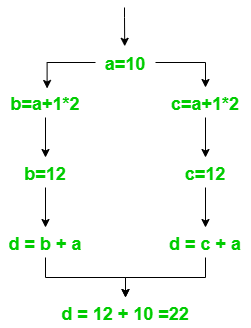Open in App
Not now

# Common Subexpression Elimination – Code optimization Technique in Compiler Design

• Last Updated : 03 Dec, 2021

Code Optimization Technique is an approach to enhance the performance of the code by either eliminating or rearranging the code lines. Code Optimization techniques are as follows:

1.  Compile-time evaluation
2.  Common Sub-expression elimination
4.  Code movement
5.  Strength reduction

### Common Sub-expression Elimination:

The expression or sub-expression that has been appeared and computed before and appears again during the computation of the code is the common sub-expression. Elimination of that sub-expression is known as Common sub-expression elimination.

The advantage of this elimination method is to make the computation faster and better by avoiding the re-computation of the expression. In addition, it utilizes memory efficiently.

### Types of common sub-expression elimination

The two types of elimination methods in common sub-expression elimination are:

1. Local Common Sub-expression elimination– It is used within a single basic block. Where a basic block is a simple code sequence that has no branches.

2. Global Common Sub-expression elimination– It is used for an entire procedure of common sub-expression elimination.

Example 1:

Before elimination

a = 10;

b = a + 1 * 2;

c = a + 1 * 2;

//’c’ has common expression as ‘b’

d = c + a;

After elimination

a = 10;

b = a + 1 * 2;

d = b + a;

Let’s understand Example 1 with a diagram:fig.: Example 1

As shown in the figure (fig.: Example 1), the result of ‘d’ would be similar with both expressions. So, we will eliminate one of the common subexpressions, as it helps in faster execution and efficient memory utilization.

Example 2:

Before elimination

```x = 11;
y = 11 * 24;
z = x * 24;
//'z' has common expression as 'y' as 'x' can be evaluated directly as done in 'y'.```

After elimination

`y = 11 * 24;`
My Personal Notes arrow_drop_up